Next: 2.2.3 Deterministic annealing Up: 2.2 Vector quantization Previous: 2.2.1 K-means

## 2.2.2 Soft-clustering

In soft-clustering, many code-book vectors compete for one pattern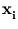. A common assignment P(j|) is the normalized Gaussian function (see, for example, Yair et al. (1992)),

 P(j|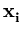) =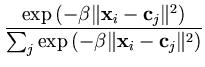. (2.14)

The parameter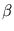controls the influence range of a patternor code-book vector. For the limit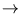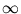, the algorithm is the same as hard-clustering. P(j|) is normalized such that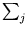P(j|) = 1 (this allows a probabilistic interpretation). Equation (2.14) can be derived using the maximum entropy principle. From all possible sets {P(j|)} that result in a given total cost E according to (2.12), the Gibbs distribution (2.14) maximizes the entropy (Rose et al., 1990). Thus, the assignment (2.14) does not rely on any assumptions about the distribution of patterns. In this physical perspective,has the role of an inverse temperature. The soft-clustering approach can be extended to an annealing process, which helps to avoid local minima (Rose et al., 1990).Next: 2.2.3 Deterministic annealing Up: 2.2 Vector quantization Previous: 2.2.1 K-means
Heiko Hoffmann
2005-03-22